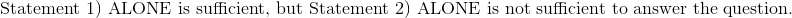# GMAT Math : DSQ: Calculating the angle for a percentage of a circle

## Example Questions

### Example Question #1 : Dsq: Calculating The Angle For A Percentage Of A Circle

Brendan's girlfriend made him a cheescake for his birthday. He eats one slice a day. What is the measure of the central angle of each slice?

I) The diameter of the cake is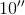.

II) Each slice is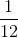of the total cake.

Statement I is sufficient to answer the question, but Statement II is not sufficient to answer the question.

Statement II is sufficient to answer the question, but Statement I is not sufficient to answer the question.

Both statements together are needed to answer the question.

Either statement alone is sufficient to answer the question.

Statement II is sufficient to answer the question, but Statement I is not sufficient to answer the question.

Explanation:

In this case we are given a circle and asked to find the angle of a portion of it.

The diameter would allow us to find many things related to the circle, but not an individual slice.

However, knowing that each slice is 1/12 of the total allows us to multiply 360 by 1/12 and find out that each slice is 30 degrees.

Therefore statement II alone is sufficient in answering the question.

### Example Question #2 : Dsq: Calculating The Angle For A Percentage Of A Circle

One slice of a pizza is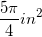. What is the central angle of one slice?

I) Each slice isof the whole pizza.

II) Each straight edge the slice is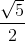inches.

Statement I is sufficient to answer the question, but Statement II is not sufficient to answer the question.

Both statements together are needed to answer the question.

Either statement alone is sufficient to answer the question.

Statement II is sufficient to answer the question, but Statement I is not sufficient to answer the question.

Either statement alone is sufficient to answer the question.

Explanation:

I) Gives us the percentage of one slice of the whole pizza. We can take 15% of 360 to find the central angle.

II) Gives us the radius of the pizza. We can use the radius to find the area of the pizza. With the total area and the area of one slice we can find the percentage of the whole and from there, the angle of one slice.

### Example Question #3 : Dsq: Calculating The Angle For A Percentage Of A Circle

Walder is at his cousin's wedding preparing to eat a slice of pie.

I) Walder's slice has a radius of half a meter

II) Walder's slice is 5% of the total pie

What is the central angle of Walder's slice?

Statement I is sufficient to answer the question, but Statement II is not sufficient to answer the question.

Statement II is sufficient to answer the question, but Statement I is not sufficient to answer the question.

Both statements are needed to answer the question.

Either statement is sufficient to answer the question.

Statement II is sufficient to answer the question, but Statement I is not sufficient to answer the question.

Explanation:

The central angle of a sector, in this case represented by the slice of pie, can be thought of as a percentage of the whole circle. Circles have 360 degrees.

Statement I gives us the radius of the circle. We could find the diameter, area, or circumference with this, but not the central angle of that slice.

Statement II gives us the percentage of the whole circle that the slice represents, 5%. We can use this to find the number of degrees in the central angle of the slice, because it will just be 5% of 360.

Thus, Statement II is sufficient, but Statement I is not.

To recap:

Walder is at his cousin's wedding preparing to eat a slice of pie.

I) Walder's slice has a radius of half a meter

II) Walder's slice is 5% of the total pie

What is the central angle of Walder's slice?

Use Statement II to find the angle. The angle must be 5% of 360: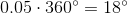### Example Question #4 : Dsq: Calculating The Angle For A Percentage Of A Circle

Alex decided to order a circular pizza. Find the angle that represents the slice of pizza he ate.

II) The slice Alex ate represented one-fifth of the total pizza.

Both statements are needed to answer the question.

Statement II is sufficient to answer the question, but statement I is not sufficient to answer the question.

Statement I is sufficient to answer the question, but statement II is not sufficient to answer the question.

Either statement is sufficient to answer the question.

Statement II is sufficient to answer the question, but statement I is not sufficient to answer the question.

Explanation:

To find the angle of a sector (in this case, that represented by the slice of pizza), we need to know with how much of the circle we are dealing.

Statement I gives us the radius of the circle. This is helpful for a lot of other things, but not finding our central angle.

Statement II tells us what portion of the pizza we are concerned with. We can multiply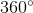by one-fifth to get the correct answer.

Using Statement II if the slice is one-fifth of the total pizza, then we can do the following to find the answer: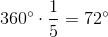Thus, Statement II is sufficient, but Statement I is not.

### Example Question #5 : Dsq: Calculating The Angle For A Percentage Of A Circle

Find the angle for the percentage of a circle.

Statement 1:  A circle diameter is 5.

Statement 2:  The sector length is.

EACH statement ALONE is sufficient.

Statement 1 ALONE is sufficient, but Statement 2 ALONE is not sufficient to answer the question.

BOTH statements TOGETHER are NOT sufficient, and additional data is needed to answer the question.

Statement 2 ALONE is sufficient, but Statement 1 ALONE is not sufficient to answer the question.

BOTH statements taken TOGETHER are sufficient to answer the question, but neither statement ALONE is sufficient.

Statement 1 ALONE is sufficient, but Statement 2 ALONE is not sufficient to answer the question.

Explanation:

The question asks to solve the angle of a percentage of a circle.

Statement 1):  A circle diameter is 5.

Statement 1) is sufficient to solve for the angle of the circle because the statement itself provides that the shape is a full circle, 360 degrees, and is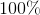of the circular sector.

Statement 2):  The sector length is.

Statement 2) does not have sufficient information to solve for the angle.  The precentage of the circle is not provided and we do not know how much of the circle will have a sector length of.  We also cannot assume that the sector is a full circle to make any further conclusions.